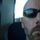cancel
Showing results for
Did you mean:New Member

## Measure with different outputs based on condition

Hi

I want to create a measure that calculates based on a condition: If Table1[Column1] = "KPI1" then Formula1 else Formula2.

The logic is very simple, but I can't get Power BI to read Table[Column] in my IF statement, it only allows me to use other measures from Table1.

Formula1 = DIVIDE(Sum(Num), Sum(Den), 0)
Formula2 = DIVIDE(Sum(Num), Average(Den), 0)

Does anyone know how to work around that?

2 ACCEPTED SOLUTIONSSuper User

``````Measure =
IF(
MAX('Table1'[Column]="KPI1",
DIVIDE(Sum(Num), Sum(Den), 0),
DIVIDE(Sum(Num), Average(Den), 0)
)``````

Become an expert!: Enterprise DNA
External Tools: MSHGQM
Latest book!:
Mastering Power BI 2nd EditionDAX is easy, CALCULATE makes DAX hard...Super User

``````MeasureName =
VAR varValue =
MAX( TableName[Field] )
RETURN
IF(
varValue = "KPI1",
DIVIDE(
SUM( Table[Num] ),
SUM( Table[Den] ),
0
),
DIVIDE(
SUM( Table[Num] ),
AVERAGE( Table[Den] ),
0
)
)
``````

You need to convert the field to a scalar value, which MAX does when used like this. MIN will give you the same result.

Did my answers help arrive at a solution? Give it a kudos by clicking the Thumbs Up!

DAX is for Analysis. Power Query is for Data Modeling

Proud to be a Super User!

MCSA: BI Reporting
4 REPLIES 4Super User

``````MeasureName =
VAR varValue =
MAX( TableName[Field] )
RETURN
IF(
varValue = "KPI1",
DIVIDE(
SUM( Table[Num] ),
SUM( Table[Den] ),
0
),
DIVIDE(
SUM( Table[Num] ),
AVERAGE( Table[Den] ),
0
)
)
``````

You need to convert the field to a scalar value, which MAX does when used like this. MIN will give you the same result.

Did my answers help arrive at a solution? Give it a kudos by clicking the Thumbs Up!

DAX is for Analysis. Power Query is for Data Modeling

Proud to be a Super User!

MCSA: BI ReportingNew Member

Works very well!!

Still intrigued why we need to work around it like that, doesn't seem like a efficient solution... PowerBI has it's problems, it shouldn't require a scalar.

Anyway, thanks so much!Super User

Everything in DAX requires it to either be scalar, column, or table,. and are not interchangable.

You cannot say IF(Table[Column] = "X", 1, 0) because in a measure it doesn't know how to process that column. It needs to be converted into a scalar value. SUM, MAX, AVERAGE, whatever.

In a Calculated Column you can do that because those have Row Context. Measures do not. It is a very hard concept to learn right off the bat, but once you do it becomes clear. Row Context and Filter Context are two completely different animals. Measures understand Filter Context, not Row Context.

There is another concept called Context Transition, and that is for another post. 😂

Did my answers help arrive at a solution? Give it a kudos by clicking the Thumbs Up!

DAX is for Analysis. Power Query is for Data Modeling

Proud to be a Super User!

MCSA: BI ReportingSuper User

``````Measure =
IF(
MAX('Table1'[Column]="KPI1",
DIVIDE(Sum(Num), Sum(Den), 0),
DIVIDE(Sum(Num), Average(Den), 0)
)``````

Become an expert!: Enterprise DNA
External Tools: MSHGQM
Latest book!:
Mastering Power BI 2nd EditionDAX is easy, CALCULATE makes DAX hard...